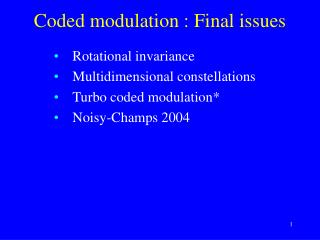DownloadDownload PresentationCoded modulation : Final issues

Coded modulation : Final issues

Télécharger la présentationCoded modulation : Final issues

- - - - - - - - - - - - - - - - - - - - - - - - - - - E N D - - - - - - - - - - - - - - - - - - - - - - - - - - -
Presentation Transcript

1. Coded modulation : Final issues • Rotational invariance • Multidimensional constellations • Turbo coded modulation* • Noisy-Champs 2004

2. Rotational invariance • Most constellations are phase symmetric: A phase shift of  will map one signal point into another, for some value of  • For example, uncoded BPSK is 180 phase invariant; uncoded QPSK 90 phase invariant • Coded modulation is in general not phase invariant, unless special care is taken • Loss of phase synchronization: • Try to resynchronize (it helps if system is not phase invariant, but still very time consuming) • Use phase invariant coded modulation scheme with differential encoding/decoding. • Will cause brief error burst at phase loss but will maintain synchronization

3. Rotational invariance: Example • Uncoded BPSK: 180 phase invariant • Coded BPSK: The coded scheme is 180 phase invariant iff, • for every code sequence v(D), it holds that v(D)1(D) is also a codeword • This in turn is true iff 1(D) is a codeword

4. Rotational invariance: Example • Uncoded QPSK: 90 phase invariant • Coded QPSK: Depends on mapping • Gray mapping • Natural mapping

5. Rotational invariance: Example V(D) = (v(1)(D), v(0)(D)) Vr(D) = (vr(1)(D), vr(0)(D)) = (v(0)(D), v(1)(D) 1(D)) Vr(D) HT(D) = u(D) ( (h(1)(D))2 (h(0)(D))2)  h(0)(D)1(D) = f(D) + h(0)(1)(D) ...is in general not = 0(D) for any choice of linear code No 90 phase invariance possible Coded QPSK with Gray mapping: Rate ½ code with • G(D) = (h(1)(D), h(0)(D)) • H(D) = (h(0)(D), h(1)(D))

6. Rotational invariance: Example V(D) = (v(1)(D), v(0)(D)) Vr(D) = (vr(1)(D), vr(0)(D)) = (v(0)(D)v(1)(D), v(0)(D)1(D)) Vr(D) HT(D) = u(D) (h(1)(D))2 h(0)(D)1(D) ...is in general not = 0(D) for any choice of linear code No 90 phase invariance possible Coded QPSK with natural mapping: Rate ½ code with • G(D) = (h(1)(D), h(0)(D)) • H(D) = (h(0)(D), h(1)(D))

7. Nonlinear parity checks • QPSK: V(D) = (v(1)(D), v(0)(D)) • v(D) = v(0)(D) + 2v(1)(D), polynomial over Z4 • vr(D) = v(D) + 1(D) (mod 4) • Parity check polynomials over Z4 : h(D) = h(1)(D) + 2h(0)(D) • Notation: • For  = 2(1)+ (0) Z4 , let []1 = (1) • Let [(D)]1 = the sequence of most significant bits in (D) • Parity check equation: [h(D) v(D) (mod 4)]1 = 0(D) • h(D) vr(D) = h(D) ( v(D) + 1(D) ) (mod 4) = h(D)v(D) + h(D)1(D)(mod 4) = h(D)v(D) + h(1)(D) (mod 4) • Satisfied iff h(1) (mod 4) = h(1)(1) + 2h(0)(1) (mod 4) = 0 • Search for parity check matrices on this form and with h(1)(D) of degree < and with no constant term

8. Nonlinear parity checks: Example • Naturally mapped QPSK, code rate 1/2 • Parity check equation: [h(D) v(D) (mod 4)]1 = [h(1)(D) v(0)(D) + 2 h(1)(D) v(1)(D) + 2 h(0)(D) v(0)(D) + 4 h(0)(D) v(1)(D) (mod 4)]1 = [h(1)(D) v(0)(D)+2(h(1)(D)v(1)(D) + h(0)(D) v(0)(D) ) (mod 4)]1 = 0(D) • Select h(1)(D) = Db+Da, 0<a<b<. • h(1)(1) = 2 so h(0)(D) must have odd weight • Parity check equation: [ (Db+Da) v(0)(D) + 2((Db+Da)v(1)(D) + h(0)(D) v(0)(D)) (mod 4)]1 = 0(D) • For ,  Z4 ,  + (mod 4) =    + 2 (  ) • PCE: [(DbDa) v(0)(D) + 2(Db v(0)(D) Da v(0)(D) (DbDa) v(1)(D) h(0)(D) v(0)(D)) (mod 4))]1 = Db v(0)(D) Da v(0)(D) (DbDa) v(1)(D) h(0)(D) v(0)(D)) (mod 4)= 0(D) • Nonlinear term: Will trellis have only 2 states?

9. Nonlinear parity checks: Example • Differential encoder • Naturally mapped QPSK, code rate 1/2 • Select h(1)(D)=D2+D, h(0)(D)=D3+D+1, H(D) = [h(1)(D)/h(0)(D),1] • Two different encoders with 8 states: • Embedded DE

10. Nonlinear parity checks Some codes:

11. Nonlinear parity checks Some codes for QAM constellations:

12. Multidimensional constellations • Instead of using only a 1-D or 2-D signal constellation S: • Send L signals from S, and consider the block of signals to be one signal point in the signal constellation SL • If S contains 2I signal points, then SL will contain 2IL points: Can send IL bits (per L symbols) • TCM system: k+1 = IL, = k/L • Advantages of multidimensional signal schemes • Wider range of spectral efficiency  • Can achieve rotational invariance with linear codes • Can use shaping to obtain further power savings • Smaller peak-to-average power ratio • Possible to obtain higher decoding speed

13. Turbo coded modulation* • Binary turbo coding achieves a considerable coding gain compared with convolutional codes • Many of the techniques for TCM can not be applied directly • Set partitioning does not make sense • Rotational invariance is difficult to achieve • Usual approach: Bit interleaving and Gray mapping • Also achieves a significant coding gain over TCM • One approach (Rosnes and Ytrehus, ICC 2004) • Good and bad bit positions in Gray mapped QAM • List of all low weight codewords • Critical positions in low weight codewords mapped to ”good” bit positions • Can achieve extremely low error floors (ML decoding)

14. Suggested exercises • 18.19-18.25

15. Suggested exercises Please acknowledge by e-mail to oyvind@ii.uib.no, in order to discuss format (length, use of projector etc.)

16. 2004 1. Joakim Knudsen 2. Sondre Rønjum 3. Uncoded